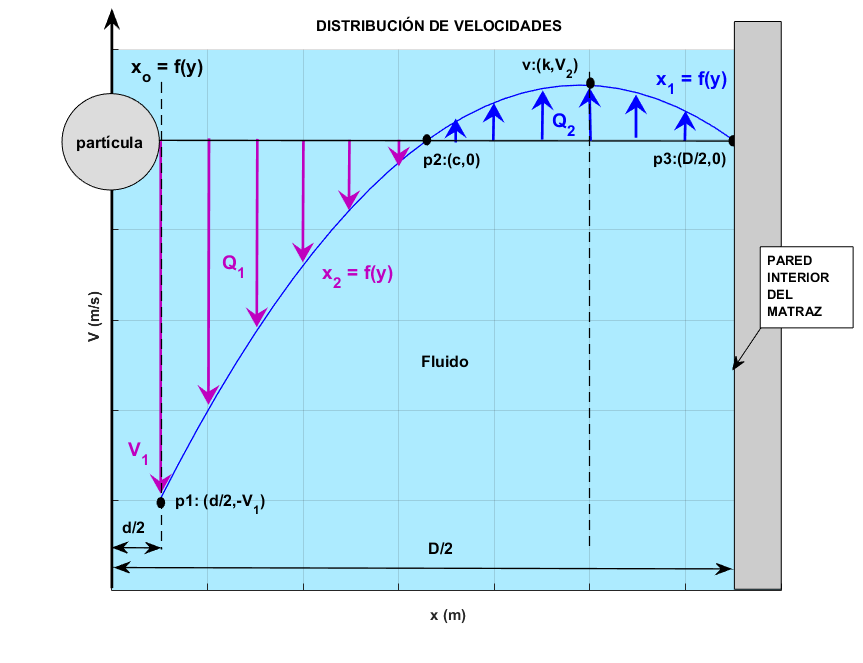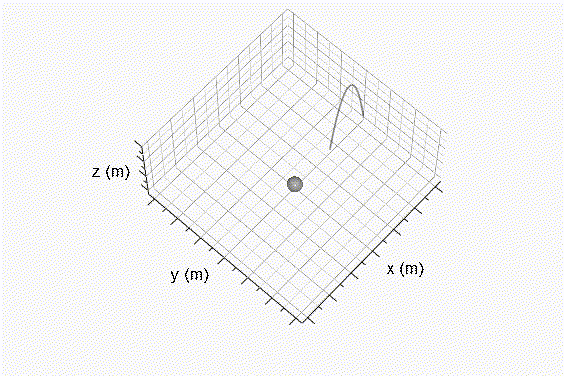Spatial distribution of steady flow around a spherical particle falling by gravity inside a water-filled flask Víctor M. Ponce and Jhonath W. Mejía 210426

 SUMMARY This article aims to answer the question asked by Professor Daryl B. Simons at Colorado State University, Fort Collins, in the mid-1970s. The problem is to determine the spatial distribution of steady flow around a spherical particle falling by gravity inside a water-filled flask. Given the diameters of the particle and the flask, we invoke the fundamental law of the conservation of mass, using volume integrals and the mean value theorem.

1.  INTRODUCTION

This article aims to answer the question asked by Professor Daryl B. Simons at Colorado State University, Fort Collins, in the mid-1970s. The problem is to determine the spatial distribution of steady flow around a spherical particle falling by gravity inside a water-filled flask. Given the diameters of the particle and the flask, we invoke the fundamental law of the conservation of mass, using volume integrals and the mean value theorem.

2.  FALL VELOCITY

The rate of fall of a sediment particle is its terminal sedimentation rate in quiescent water. The rate of fall is a function of the size, shape, and specific gravity of the particle and the specific gravity and viscosity of the water. For small particles, their shape can approximate that of a sphere; then, the rate of fall is expressed as follows (Ponce, 1989):

 4      g d     γs  -  γ w  =  [ ___  ______  ________ ] 1/2              3      CD          γ (1)

in which w = fall velocity; g = gravitational acceleration; d = particle diameter; γ = specific weight of water; γs = specific weight of the sediment particles, and CD = resistance coefficient (friction). This equation can be solved iteratively with the online calculator onlinefallvelocity.

3. VELOCITY DISTRIBUTION

The velocity distribution in the space between the particle and the inner wall of the flask has the following characteristics (Fig. 1):

• The center of the particle is at the origin of coordinates.

• The flow close to the particle is given by Q1, while the flow close to the interior wall of the flask is given by Q2; these flows are equal due to the law of conservation of mass.

• The value V1 is the fall velocity of the particle.

• The value V2 is the maximum upward flow velocity.

• The boundary condition on the inner wall of the flask is the no-slip condition, that is Vo = 0.

• The value of c is the point of intersection between the velocity distribution with the x-axis, and it is where the equilibrium of the two flows Q1 and Q2 is established.Fig. 1  Velocity distribution in the space between the particle and the inner wall of the flask.

From Figure 1, the following relationships may be established. The canonical equation of a parabola with vertix at (k,V2) is defined as follows:

 (y - V2) = 4P (x - k) 2 (2)

From Equation 2, solving for x:

 ( y - V2) x1 = k + [ ________ ]1/2                     4P (3)

 (y - V2) x2 = k - [ ________ ]1/2                     4P (4)

Evaluating Eq. 2 at p1:(0.5d, -V1) and solving for 4P :

 - (V1 + V2) 4P = ____________            (0.5d - k)2 (5)

Evaluating Eq. 2 at p2:(c, 0) and solving for c :

 -V2 c = k - [ ____ ]1/2                4P (6)

Evaluating Eq. 2 at p3:(0.5D, 0) and solving for 4P :

 -V2 4P = ___________            (0.5D - k)2 (7)

Equating Eqs. 5 and 7:

 - (V1 + V2)          - V2 ____________ = ____________    (0.5d - k)2       (0.5D - k)2 (8)

 (V1 + V2)        (0.5d - k)2 __________ = ___________       V2            (0.5D - k)2 (9)

From Eq. 9, solving for V1:

 (0.5d - k)2 V1 = V2 [ ___________ - 1 ]                  (0.5D - k)2 (10)

For RV = (V1 + V2) /V2 and RD = (0.5d - k)2/(0.5D - k)2, Eqs. 9 and 10 are redefined as follows:

 Rv = RD (11)

 V1 = V2 ( RD - 1 ) (12)

Substituting Eq. 7 in Eqs. 3 and 4, and simplifying:

 y x1 = k + (0.5D - k) [ 1 - ____ ]1/2                                        V2 (13)

 y x2 = k - (0.5D - k) [ 1 - ____ ]1/2                                       V2 (14)

4.  CONSERVATION OF MASS

By applying the principle of conservation of mass in Fig. 1, the following is established:

 Q1  -  Q2  =  0 (15)

Rewriting Eq. 15 in terms of area and mean velocity:

 Vm1 A1  =  Vm2 A2 (16)

in which A1 = π [c2 - (0.5d)2] /4, flow area of the inner ring; and A2 = π [(0.5D)2 - c2] /4, flow area of the outer ring. From Fig. 1 and the mean value theorem for volume integrals, the mean velocity Vm1 is defined as follows:

 ∀v1 Vm1  =  _____               A1 (17)

in which ∀V1 = Volume 1. The mean velocity Vm2 is defined as follows:

 ∀v2 Vm2  =  _____               A2 (18)

in which ∀V2 = Volume 2. Replacing Eqs. 17 and 18 in Eq. 16:

 ∀v1                ∀v2 _____  A1  =  _____  A2   A1                 A2 (19)

and simplifying:

 ∀v1  =  ∀v2 (20)

Equation 20 represents the equilibrium condition that exists between the flows Q1 and Q2 as defined in Eq. 15, in terms of volumes.

Solving ∀V1 using the integral of the volume of a solid of revolution generated by the curve x2, given Eq. 14:

 0                                            y ∀v1  =  π  ∫ { [ k  -  (0.5D - k) ( 1  -  ____ )1/2 ] 2  -  (0.5D) 2 } dy                   -V1                                           V2 (21)

 (0.5D - k) 2           y                      4k (0.5D - k) V 2       y                                       0 ∀v1  =  π [ k 2y  -  _________ V 2 ( ____  -  1 ) 2  +  _______________ ( ____  -  1 ) 3/2  -  (0.5D) 2y ]                                    2                   V2                                3                  V2                                     -V1 (22)

Taking θ1 = (0.5D - k) and θ2 = (0.5D), and replacing in Eq. 22:

 (θ1) 2             y                       4k (θ1) V 2         y                                    0 ∀v1  =  π [ k 2y  -  _____ V 2 ( ____  -  1 ) 2  +  ___________ ( ____  -  1 ) 3/2  -  (θ2) 2y ]                                 2               V2                              3              V2                                  -V1 (23)

Replacing the limits (0, -V1) for y in Eq. 23, and simplifying:

 -θ12V2        4k θ1V2                     θ12V2       V1 + V2             4k θ1V2       V1 + V2  ∀v1  =  π [ _______  +  ________  +  V1k 2  +  ______ ( ________ ) 2  -  ________ ( ________ ) 3/2  +  (θ2) 2V1 ]                       2                 3                             2              V2                       3              V2 (24)

Taking RV = (V1 + V2) /V2 and replacing in Eq. 24:

 -θ12V2         4k θ1V2                      θ12V2             4k θ1V2        ∀v1  =  π [ _______  +  ________  +  V1k 2  +  ______ Rv2  -  ________ Rv3/2  +  (θ2) 2V1 ]                       2                 3                               2                     3 (25)

Simplifying:

 -θ12V2                              4k θ1V2                      ∀v1  =  π [ _______ ( 1  -  Rv2 )  +  __________ ( 1  -  Rv3/2 )  +  V1 (k 2  +  θ2 2 ) ]                       2                                      3 (26)

Replacing Eqs. 11 and 12 in Eq. 26:

 -θ12V2                               4k θ1V2                      ∀v1  =  π [ _________ ( 1  -  RD2 )  +  _________ ( 1  -  RD3/2 )  +  V2 ( RD - 1 ) (k 2  +  θ2 2 ) ]                        2                                        3 (27)

 -θ12                            4k θ1                      ∀v1  =  π V2 [ _____ ( 1  -  RD2 )  +  ______ ( 1  -  RD3/2 )  -  ( 1 - RD ) (k 2  +  θ2 2 ) ]                          2                                 3 (28)Fig. 2  Surface of revolution generated below the origin of coordinates.

Solving ∀V2 using the integral of the volume of a solid of revolution generated by the curves x1 and x2, given Eqs. 13 and 14, respectively:

 ∀v2  =  π  ∫ { [ x1(y) ]2  -  [ x2(y) ]2 } dy (29)

Solving the integral for x1(y):

 V2                                       y  ∫ [ x1(y) ] 2dy  =   ∫ [ k  +  (0.5D - k) ( 1  -  ____ )1/2 ] dy                             0                                        V2 (30)

 (0.5D - k) 2            y                       4k (0.5D - k) V 2       y                     V2  ∫ [ x1(y) ] 2dy  =   [ k 2y  -  ___________ V 2 ( ____  -  1 ) 2  -  _______________ ( ____  -  1 ) 3/2 ]                                                     2                   V2                               3                     V2                    0 (31)

Replacing the limits (V2, 0) for y in Eq. 31 and simplifying:

 (0.5D - k) 2        4k (0.5D - k)   ∫ [ x1(y) ] 2dy  =  V2 [ k 2  +  ___________  +  _____________ ]                                                      2                       3 (32)

Solving the integral for x2(y):

 V2                                       y  ∫ [ x2(y) ] 2dy  =  ∫ [ k  -  (0.5D - k) ( 1  -  ____ )1/2 ] dy                              0                                        V2 (33)

 (0.5D - k) 2           y                      4k (0.5D - k) V 2        y                     V2  ∫ [ x2(y) ] 2dy  =  [ k 2y  -  __________ V 2 ( ____  -  1 ) 2  +  ________________ ( ____  -  1 ) 3/2 ]                                                   2                   V2                                 3                  V2                      0 (34)

Replacing the limits (V2, 0) for y in Eq. 34 and simplifying:

 (0.5D - k) 2        4k (0.5D - k)   ∫ [ x2(y) ] 2dy  =  - V2 [ k 2  +  ___________  -  _____________ ]                                                           2                        3 (35)

Replacing Eqs. 32 and 35 in Eq. 29:

 (0.5D - k) 2       4k (0.5D - k)                             (0.5D - k) 2       4k (0.5D - k)  ∀v2  =  π V2 [ k 2  +  _________  +  ______________ ] -  π V2 [ k 2  +  _________  -  ______________ ]                                       2                        3                                               2                       3 (36)

 8k (0.5D - k)  ∀v2  =  π V2 [  ______________ ]                                   2 (37)

Taking θ1 = (0.5D - k), and replacing in Eq. 37:

 8k θ1  ∀v2  =  π V2 [  _______ ]                            2 (38)Fig. 3   Surface of revolution generated above the origin of coordinates.

Replacing Eqs. 28 and 38 in Eq. 20:

 -θ12                             4k θ1                                                                                          8k θ1 π V2 [ ____ ( 1  -  RD2 )  +  ________ ( 1  -  RD3/2 )  -  ( 1 - RD ) (k 2  +  θ2 2 ) ]  =  π V2 [  _______ ]               2                                  3                                                                                                 2 (39)

Simplifying Eq. 39:

 -θ12                            4k θ1  [ ____ ( 1  -  RD2 )  +  ______ ( 1  -  RD3/2 )  -  ( 1 - RD ) (k 2  +  θ2 2 ) ]  =  4k θ1         2                                 3 (40)

Rewriting Eq. 40 in terms of d, D, and k:

 -(0.5D - k)2       (0.5d - k)4       4k (0.5D - k)     (0.5d - k)3              (0.5d - k)2  __________ (1 - _______ )  +  ___________ (1 - ________ )  -  (1 - ________ ) (k 2 + (0.5D)2)  =  4k (0.5D - k)         2               (0.5D - k)4               3               (0.5D - k)3              (0.5D - k)2 (41)

Equation 41 has as input parameters: d = particle diameter, D = internal diameter of the flask, and as output parameter k = vertix of the parabola in the velocity profile (Fig. 1). Since this equation is implicit for k, an iterative method is used to solve it. Once k is obtained, Eq. 10 is used to calculate V2:

 (0.5d - k)2 V2 = V1 [ ____________ - 1 ] -1                   (0.5D - k)2 (42)

5. EXAMPLE

Calculate the velocity distribution around a spherical particle of diameter d = 1.0 mm, which falls into quiescent water in a flask of internal diameter D = 6.5 cm. Consider: T = 20 °C; γs = 2.65 gr/cm3; γ = 1.0 gr/cm3, and g = 9.81 m/s2.

1. Calculate the fall velocity of the particle, using the program onlinefallvelocity:

V1  =  0.155 m/s

2. Calculate k using Eq. 41:

k  =   0.02438 m

3. Calculate V2 using Eq. 42:

[(0.001/2) - 0.02438] 2
V2  =  0.155 [ ________________________ - 1 ] -1
[(0.065/2) - 0.02438] 2

V2  =  0.0203 m/s

4. Calculate 4P using Eq. 7:

- 0.0203
4P  =  _______________________
[(0.065/2) - 0.02438] 2

4P  =  - 3.0732

5. Calculate c using Eq. 6:

- 0.0203
c  =  0.02438 - [ ___________ ]1/2
- 3.0732

c  =  0.01626 m

6. CONCLUSIONS

The spatial distribution of the steady flow around a spherical particle falling in a flask filled with quiescent water has been calculated. Given the diameters of the particle and the flask, and using the fundamental law of conservation of mass, volume integrals, and the mean value theorem, the coordinates of the point p2 are calculated, which separates the upward flow, close to the inner wall of the flask, from the downward flow, adjacent to the particle.

REFERENCES

Ponce, V. M. 1989. Engineering Hydrology, Principles and Practices, Prentice Hall.

Piskunov, N. 1969. Differential and integral calculus, Mir Editorial.

 210506 21:00